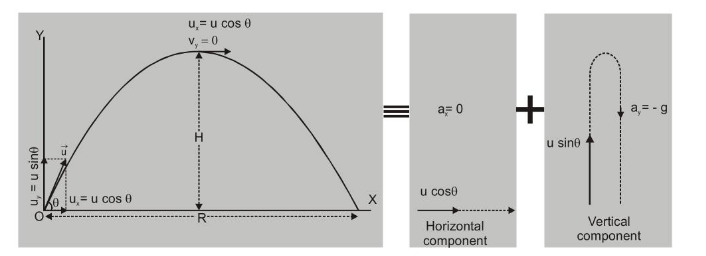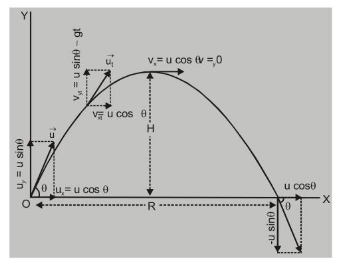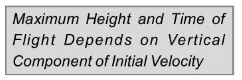Most Affordable JEE | NEET | 8,9,10 Preparation by Kota's Top IITian Doctor Faculties

# What is Oblique Projectile Motion?## Oblique Projectile Motion

Consider the motion of a bullet that is fired from a gun so that its initial velocity $\vec{u}$ makes an angle $\theta$ with the horizontal direction. Let us take $X$-axis along the ground and $Y$-axis along vertical.

$\vec{u}$ can be resolved as

$\mathrm{u}_{\mathrm{x}}=\mathrm{u} \cos \theta \quad$ (along horizontal)

$\& u_{y}=u \sin \theta$ (along vertical)

The motion of bullet can be resolved into horizontal and vertical motion.

(i) In horizontal direction there is no acc. so it moves with constant velocity $v_{x}=u_{x}=u \cos \theta$

So distance traversed in time $t$ is $x=u_{x} t$ or $x=(u \cos \theta) t$ or $t=\frac{x}{u \cos \theta}$.The motion in the vertical direction is the same as that of a ball thrown upward with an initial velocity

$u_{y}=u \sin \theta$ and $a c c=-g$ (downward).

So at time $t$ vertical component of velocity $v_{y}=u_{y}-g t=u \sin \theta-g t$    ...(ii)

Displacement along y direction $y=(u \sin \theta) t-\frac{1}{2} g t^{2}$    ...(iii)

Substituting the value of $t$ from eqn. (i) in eqn. (iii)

we get $y=(u \sin \theta)\left(\frac{x}{u \cos \theta}\right)-\frac{1}{2} g\left(\frac{x}{u \cos \theta}\right)^{2}$

or $y=x \tan \theta-\frac{g}{2 u^{2} \cos ^{2} \theta} \cdot x^{2}$. This is eqn. of parabola.

The trajectory of projectile is parabolic

The projectile will rise to maximum height $\mathrm{H}$ (where $\mathrm{v}_{\mathrm{x}}=\mathrm{u} \cos \theta, \mathrm{v}_{\mathrm{y}}=0$ ) and then move down again to reach the ground at a distance $R$ from origin.

Setting $x=R$ and $y=0$ (since projectile reaches ground again)$O=R \tan \theta-\frac{g}{2 u^{2} \cos ^{2} \theta} \cdot R^{2}$

We get $R=\frac{2 u^{2} \cos ^{2} \theta}{g} \times \frac{\sin \theta}{\cos \theta}$

or $\quad \mathrm{R}=\frac{2 \mathrm{u}^{2}}{\mathrm{~g}} \cdot \sin \theta \cos \theta$

or Range  $\mathrm{R}=\frac{\mathrm{u}^{2} \sin 2 \theta}{\mathrm{g}}$

If time for upward journey is $t$

at highest point $\quad v_{y}=0$ so

$0=(u \sin \theta)-g t \quad\left(v_{y}=u_{y}-g t\right)$

or $\quad \mathrm{t}=\frac{\mathrm{u} \sin \theta}{\mathrm{g}}$

$\therefore \quad \mathrm{T}=2 \mathrm{t}$    (it will take same time for downward journey)

$\therefore \quad \mathrm{T}=\frac{2 \mathrm{u} \sin \theta}{\mathrm{g}}$    Time of flight

At the highest point $y=H$ and $v_{y}=0$

So that $H=\frac{u_{y}^{2}}{2 g}$    $\left[v_{y}^{2}=u_{y}^{2}-2 g y\right]$or $\quad \mathrm{H}=\frac{\mathrm{u}^{2} \sin ^{2} \theta}{2 \mathrm{~g}} \quad$ Maximum Height

we can also determine $\mathrm{R}$ as follows

$x=u_{x} t$

so $\quad \mathrm{R}=\mathrm{u}_{\mathrm{x}} . \mathrm{T}$

$=(u \cos \theta)\left(\frac{2 u \sin \theta}{g}\right)$

or $\quad \mathrm{R}=\frac{\mathrm{u}^{2} \sin 2 \theta}{\mathrm{g}}$

velocity at time $t \vec{v}_{t}=v_{x t} \hat{i}+v_{y t} \hat{j}=(u \cos \theta) \hat{i}+(u \sin \theta-g t) \hat{j}$

$v=\sqrt{u^{2} \cos ^{2} \theta+(u \sin \theta-g t)^{2}}$

Note :

(i) Alternative eq $^{n}$. of trajectory $y=x \tan \theta\left(1-\frac{x}{R}\right)$ where $R=\frac{2 u^{2} \sin \theta \cos \theta}{g}$

(ii) Vertical component of velocity $v_{y}=0$, when particle is at the highest point of trajectory.

(iii) Linear momentum at highest point $=m u \cos \theta$ is in horizontal direction.

(iv) Vertical component of velocity is +ive when particle is moving up.

(v) Vertical component of velocity is -ive when particle is moving down.

(vi) Resultant velocity of particle at time $t v=\sqrt{v_{x}^{2}+v_{y}^{2}}$ at an angle $\phi=\tan ^{-1}\left(\frac{v_{y}}{v_{x}}\right)$.

(vii) Displacement from origin, $s=\sqrt{x^{2}+y^{2}}$